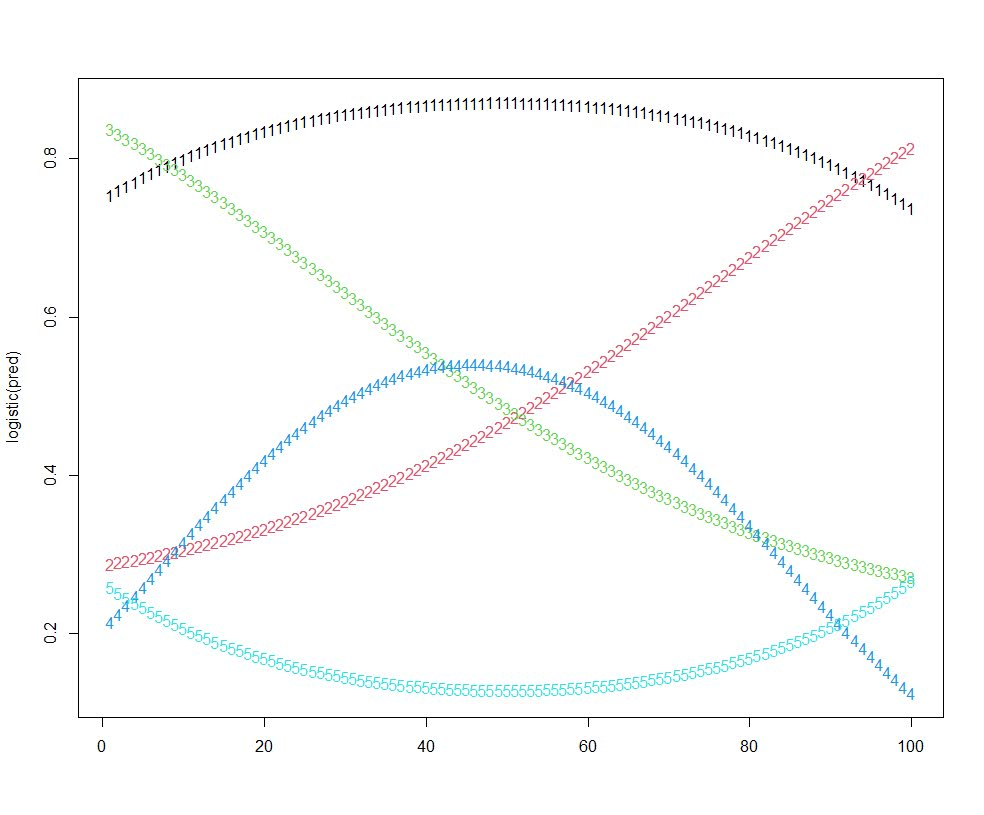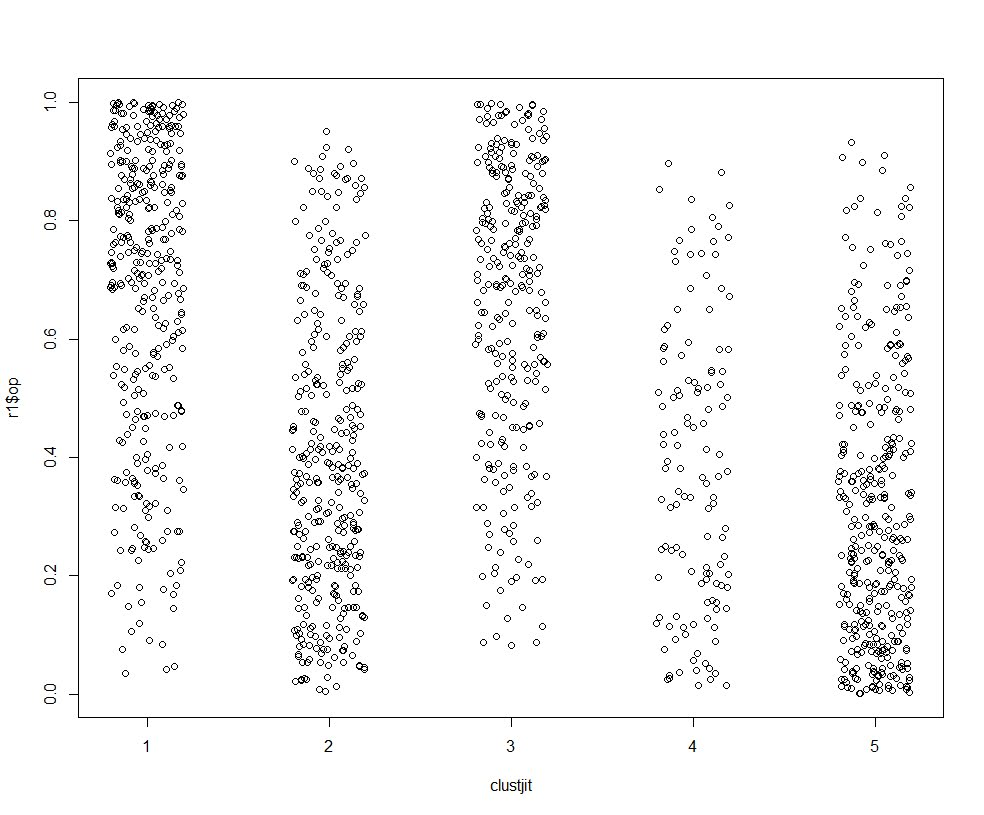# State dependence and trajectory models

I am currently reviewing a paper that uses group based trajectory models (GBTM) – and to start this isn’t a critique of the paper. GBTM I think is a very useful descriptive tool (how this paper I am reading mostly uses it), and can be helpful in some predictive contexts as well.

It is much more difficult though to attribute a causal framework to those trajectories though. First, my favorite paper on this topic is Distinguishing facts and artifacts in group-based modeling (Skardhamar, 2010). Torbjørn in that paper simulates random data (not dissimilar to what I do here, but a few more complicated factors), and shows that purely random data will still result in GBTM identifying trajectories. You can go the other way as well, I have a blog post where I simulate actual latent trajectories and GBTM recovers them, and another example where fit stats clearly show a random effects continuous model is better for a different simulation. In real data though we don’t know the true model like these simulations, so we can only be reasonably skeptical that the trajectories we uncover really represent latent classes.

In particular, the paper I was reading is looking at a binary outcome, so you just observe a bunch of 0s and 1s over the time period. So given the limited domain, it is difficult to uncover really wild looking curves. They ended up finding a set of curves that although meet all the good fit stats, pretty much cover the domain of possibilities – one starting high an linearly sloping down, one starting low and sloping up, one flat high, one flat low, and a single curved up slope.

So often in criminology we interpret these latent trajectories as population heterogeneity – people on different curves are fundamentally different (e.g. Moffitt’s taxonomy for offending trajectories). But there are other underlying data generating processes that can result in similar trajectories – especially over a limited domain of 0/1 data.

Here I figured the underlying data the paper I am reviewing is subject to very strong state dependence – your value at t-1 is very strongly correlated to t. So here I simulate data in R, and use the flexmix package to fit the latent trajectories.

First, I simulate 1500 people over 15 time points. I assign them an original probability estimate uniformly, then I generate 15 0/1 observations, updating that probability slightly over time with an auto-correlation of 0.9. (Simulations are based on the logit scale, but then backed out into 0/1s.)

``````# R Code simulating state dependence 0/1
# data
library("flexmix")
set.seed(10)

# logit and inverse function
logistic <- function(x){1/(1+exp(-x))}
logit <- function(x){log(x/(1-x))}

# generate uniform probabilities
n <- 1500
orig_prob <- runif(n)

# translate to logits
ol <- logit(orig_prob)
df <- data.frame(id=1:n,op=orig_prob,ol)

# generate auto-correlated data for n = 10
auto_corr <- 0.90
tp <- 15
vl <- paste0('v',1:tp)
vc <- var(ol) #baseline variance, keep equal

for (v in vl){
# updated logit
rsd <- sqrt(vc - vc*(auto_corr^2))
ol <- ol*0.9 + rnorm(n,0,rsd)
# observed outcome
df[,v] <- rbinom(n,1,logistic(ol))
}``````

This generates the data in wide format, so I reshape to long format needed to fit the models using flexmix, and I by default choose 5 trajectories (same as chosen in the paper I am reviewing).

``````# reshape wide to long
ld <- reshape(df, idvar="id", direction="long",
varying = list(vl))

# fit traj model for binary outcomes
mod <- flexmix(v1 ~ time + I(time^2) | id,
model = FLXMRmultinom(),
data=ld, k=5)

rm <- refit(mod)
summary(rm)``````

Now I create smooth curves over the period to plot. I am lazy here, the X axis should actually be 1-15 (I simulated 15 discrete time points).

``````tc <- summary(rm)@components[]
pd <- data.frame(c=1,t=seq(1,tp,length.out=100))
pd\$tsq <- pd\$t^2

co <- matrix(-999,nrow=3,ncol=5)

for (i in 1:5){
vlab <- paste0('pred',i)
co[,i] <- tc[[i]][,1]
}

pred <- as.matrix(pd) %*% co

# plot on probability scale
matplot(logistic(pred))``````These are quite similar to the curves for the paper I am reviewing, a consistent low probability (5), and a consistent high (1), a downward mostly linear slope (3), and an upward linear slope (2), and then one parabola concave down (4) (in the paper they had one concave up).

I figured the initial probability I assigned would highly impact the curve the model assigned a person to in this simulation. It ends up being more spread out than I expected though.

``````# distribution of classes vs original probability
ld\$clus <- clusters(mod)
r1 <- ld[ld\$time == 1,]
clustjit <- r1\$clus + runif(n,-0.2,0.2)
plot(clustjit,r1\$op) # more spread out than I thought``````So there is some tendency for each trajectory to be correlated based on the original probability, but it isn’t that strong.

If we look at the average max posterior probabilities, they are OK minus the parabola group 4.

``````# average posterior probability
pp <- data.frame(posterior(mod))
ld\$pp <- pp[cbind(1:(n*tp),ld\$clus)]
r1 <- ld[ld\$time == 1,]
aggregate(pp ~ clus, data = r1, mean)
#   clus        pp
# 1    1 0.8923801
# 2    2 0.7903938
# 3    3 0.7535281
# 4    4 0.6380946
# 5    5 0.8419221``````

The paper I am reviewing has much higher APPs for each group, so maybe they are really representing pop heterogeneity instead of continuous state dependence, it is just really hard with such observational data to tell the difference.